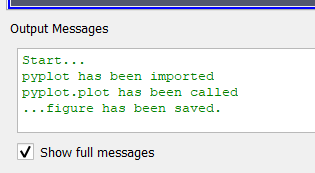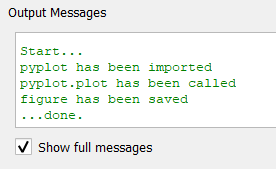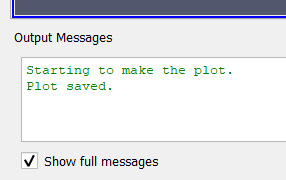# "Output messages" window stop working after running a macro that uses Matplotlib (MacOS)

Hi. I have a Python macro that outputs some information about the data being visualized in the “Output Messages”. window. This macro also has a function that makes a plot using Matplotlib and saves it as a PNG file. However, after this function runs, the output messages windows does not display anything else. It is almost like if the default IO had been redirected to something else. Does anyone know a possible solution to get the output messages windows back to work? Here is a short example that illustrates the problem:

``````import matplotlib.pyplot as plt
print("Starting to make the plot.")
#fig, ax = plt.subplots(); plt.close("all")
print("Plot saved.")
``````

Nothing is shown in output messages window if I uncomment the line.

Hi Matheus,

I tried to use matplotlib.pyplot inside a macro for ParaView. The macro:

print(‘Start…’)
import matplotlib.pyplot as plt
print(‘pyplot has been imported’)
x = [1,2,3]
y = [5,3,7]
plt.plot(x, y)
print(‘pyplot.plot has been called’)
plt.savefig(r’C:\P\testgfig.png’)
print(’…figure has been saved.’)

After running it, ‘output messages’ window shows all the printed lines:and I have a plot in png file:

In case of using subplots, it also works:

print(‘Start…’)
import matplotlib.pyplot as plt
print(‘pyplot has been imported’)
x = [1,2,3]
y = [5,3,7]
fig, ax = plt.subplots(nrows=1, ncols=1)
ax.plot(x, y)
print(‘pyplot.plot has been called’)
fig.savefig(r’C:\P\testgfig2.png’)
print(‘figure has been saved’)
plt.close(fig)
print(’…done.’)And I can see the same picture saved on a disk.
With ‘plt.close(“all”)’, the output is the same.

Hope this is a solution for you.

Best Regards,
Pavel

Hi Pavel. Thank you for looking at this.

I used plt.close(“all”) just to make sure all plots are closed when I created them inside a loop. It is not necessary in this example and I still see the problem when I get rid of it.

I tried the version of code you posted first and I still have problem. Nothing is shown and no file is saved. I am running Paraview 5.10.0-RC2 on Mac OS 11.6. I see you are running Windows. Do you think the OS is the source of problem here?

I am using ParaView 5.9.1 (yes, on Windows).
I tried to run your script exactly:

import matplotlib.pyplot as plt
print(“Starting to make the plot.”)
fig, ax = plt.subplots(); plt.close(“all”)
print(“Plot saved.”)

and it works:I’m not sure that the problem is in MacOS. Could you please check the path to the png file which you specify for savefig()? Or provide the full script?

Best Regards,
Pavel

This is the code I am running now:

``````print("Start…")
import matplotlib.pyplot as plt
print("pyplot has been imported")
x = [1,2,3]
y = [5,3,7]
plt.plot(x, y)
print("pyplot.plot has been called")
plt.savefig("/Users/viana/Desktop/viz/testgfig.png")
print("…figure has been saved.")
``````

Nothing is shown and no file has been saved. Yes, the path is correct.

Looks good. I’m sorry for stupid question: did you ‘Import new macro…’ first, and then click on the name of the loaded macro in the list below on the same menu panel?

Yes, this is what I do. If I try a second time, then Paraview crashes on me. However, this second time the file was saved. Nothing is shown in “Output Messages” window both times.

Thanks. And if you do not use matplotlib.pyplot at all, can you see some output in “Output Messages” window?

Yes. Everything fine if I don’t use Matplotlib.

Ok. We can check matplotlib version, but I’m not sure it will help. I think we need help from someone from Kitware.

This is what I have:

``````>>> matplotlib.__version__
'3.2.1'
``````

Let me test another version of Paraview.

Everything works fine on Paraview 5.9.1. Maybe you could try to run Paraview 5.10 and see if you notice the same problem on Windows?

Yes, I can try the same script for PV 5.10.

I checked. All the previous scripts work fine in PV 5.10.0 (on Windows). Previously you mentioned Paraview 5.10.0-RC2. Maybe downloading and using stable version 5.10.0 is the solution for your issue?

Good point. I will try that at some point today and post the result here.
Best,
Matheus

Same problem using stable PV 5.10.0. So annoying sigh… The rendering looks so much better for me in PV 5.10 but now bc of this bug I am stuck with PV 5.9. I hope someone else have other ideas…

By the way, I have an issue with importing matplotlib using macro or Python Shell in ParaView 5.9.1 installed on my other machine with Ubuntu. Outside ParaView it does work.
(matplotlib version 3.5.1).

What issue is that?

I just can not import matplotlib module inside Python shell or using macro. I think in my case smth wrong with environment settings regarding to Python. Tomorrow I’ll have an access to that machine and be able to put an error message here.

Edited:

Hi @mwestphal. Could you please take a look at this issue?